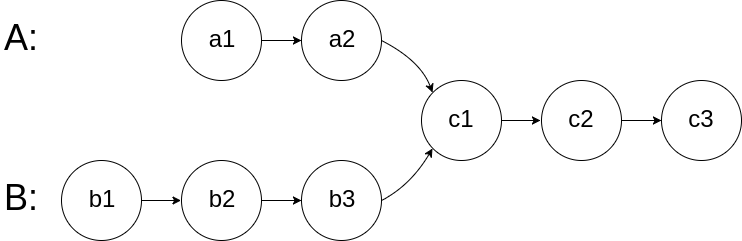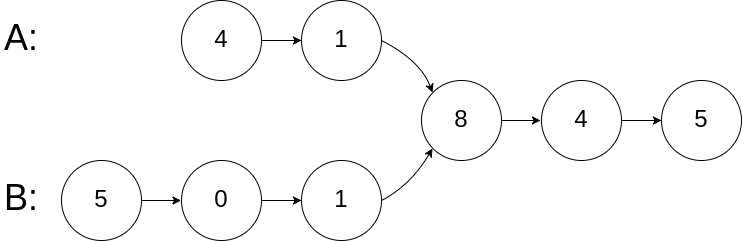## 1.题目描述## 2.解答

/**
* public class ListNode {
*     int val;
*     ListNode next;
*     ListNode(int x) {
*         val = x;
*         next = null;
*     }
* }
*/
public class Solution {
int lenA = 0,lenB = 0;
//分别遍历两个链表，求出二者长度的差值
while(temp != null){
lenA++;
temp = temp.next;
}
while(temp != null){
lenB++;
temp = temp.next;
}
//设置变量，尽量不要移动头指针
int diff = lenA - lenB;
//让比较长的链表先走出差值
while(true){
if(diff > 0){
p1 = p1.next;
diff--;
}
if(diff < 0){
p2 = p2.next;
diff++;
}
if(diff == 0)
break;
}
//当两个节点满足 == 时，返回
while(p1 != p2){
p1 = p1.next;
p2 = p2.next;
}
return p1;
}
}


• 两个链表都从第一个节点，每次以相同的速度向后面遍历，
• 如果最终两个链表存在相交的话，那么从第一个相交节点开始，两个链表一定是手挽手，肩并肩的一起往后面走的，返回第一个即可，
• 如果不相交，那么两个指针从头到尾，以相同的速度，走了同样远的路程，最后同时为null，返回null即可

/**
* public class ListNode {
*     int val;
*     ListNode next;
*     ListNode(int x) {
*         val = x;
*         next = null;
*     }
* }
*/
public class Solution {
while(p1 != p2){
p1 = p1 == null ? headB : p1.next;
p2 = p2 == null ? headA : p2.next;
}
return p1;
}
}


• 创建一个HashMap，键为节点的值，值为节点new HashMap<Integer,ListNode>()

• 将任意一个链表中的节点全部存入HashMap中

• 遍历另外一个链表，将每个节点和HashMap进行比较，如果HashMap中包含与当前节点Key相等的节点，那么再比较两个节点时候地址相等，也就是进行==比较

• 进行上述两次比较之后，这样就可以确保两个节点是同一个

/**
* public class ListNode {
*     int val;
*     ListNode next;
*     ListNode(int x) {
*         val = x;
*         next = null;
*     }
* }
*/
public class Solution {
HashMap<Integer,ListNode> map = new HashMap<Integer,ListNode>();
while(p1 != null){
map.put(p1.val,p1);
p1 = p1.next;
}